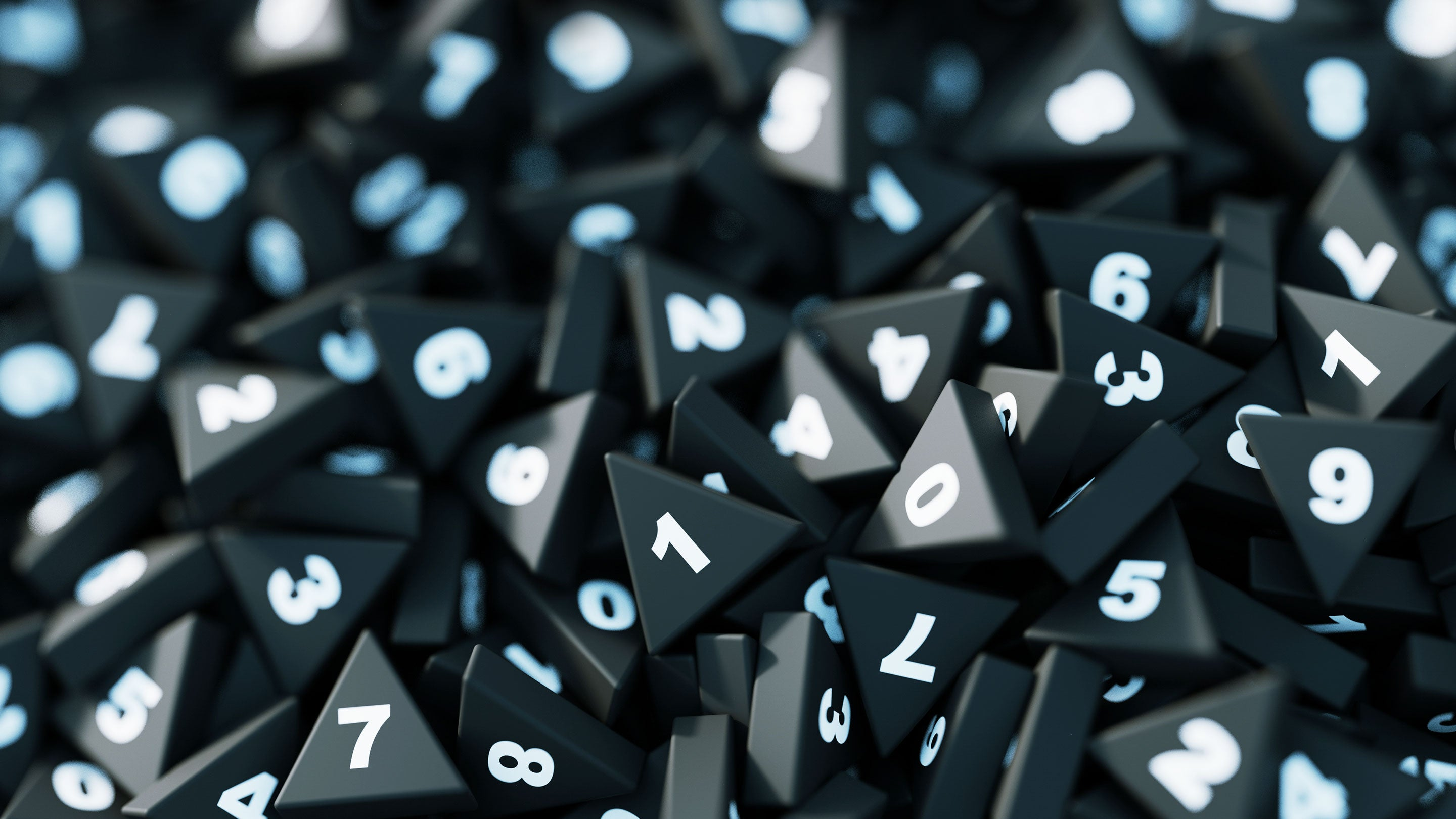# How Randomness Can Make Math Easier

Randomness would seem to make a mathematical statement harder to prove. In fact, it often does the opposite.Explore

Of all the tools available to the mathematician, randomness would seem to offer little benefit. Math traffics in logic and rigor. Its broad goals are to find order and structure in a vast sea of objects. It’s precisely because the mathematical world isn’t random that the whole enterprise of mathematics is possible.

Yet the recent Quanta article “Random Surfaces Hide an Intricate Order” concerned a new proof in which randomness made all the difference. The result involves checkerboard-like patterns drawn atop geometric spaces that are built at random. The authors of the proof found that the randomness in the geometric space made the checkerboard patterns easier to describe. “It’s a bit surprising that adding randomness enables you to do more than you can” without it, said Nicolas Curien, a mathematician at Paris-Sud University and a co-author of the work.

As it turns out, randomness is helpful in mathematics in many ways.

For example, mathematicians often want to prove that an object with a certain property exists, such as a geometric object that exhibits particular symmetries. The most direct way to solve these existence problems is to find an example of an object with the properties you’re after. But good luck with that. “It can be difficult to exhibit one particular object with the property in question,” said Martin Hairer, a Fields Medal winner whose work involves random processes.

If a direct assault on the problem is unlikely to succeed, you might try a flank attack. For example, you could show that if you were to consider all objects of a certain type, and then select one of them at random, there’s a greater than 0% chance that you’ll select one with the required property. This “probabilistic method” was pioneered by the mathematician Paul Erdős.

Randomness can also be used to find a path to a nonrandom solution. This was the case in the recent proof about checkerboard-like patterns on a grid. The researchers were interested in a process called percolation, in which you want to know under what conditions it’s possible to travel on points of only one color from one side of the grid to another.

When you draw such a path according to deterministic rules—along the rigidly determined lines of a regular grid—each subsequent step in the path is bound by every step in the path that’s come before it. In the case of a complicated grid, this requirement is something of a burden. It’s similar to how the first few pieces in a Tetris puzzle are easy to place—you can put them wherever you want—but later ones are much harder, because they have to conform to all the pieces you’ve already set down.

Yet when your path proceeds at random, you don’t have to worry about your past steps. Each step is, in a sense, as free as the first one: Just flip a coin to decide where to go next.

Mathematicians try to exploit this fact. There’s a conjectural relationship, known as the KPZ formula, that tells mathematicians how to convert a result about the random grid into a result for the deterministic one, or vice versa. “In theory it means you’re free to compute on either” the random or deterministic side, said Olivier Bernardi, a mathematician at Brandeis University and a co-author of the recent paper. This new work is consistent with previous (much harder to prove) results about percolation on a regular grid, validating the KPZ formula.

If mathematics were easier, mathematicians might not need to resort to randomness. But most important mathematical questions are too hard for mathematicians to answer directly. “It’s something that may be obvious, but is good to remember, that most of the time if you state a problem in math or theoretical physics, it’s impossible,” said Paul Bourgade, a mathematician at New York University. “We just don’t have the tools to solve it.” In some of these situations, randomness loosens things up just enough to make a solution possible.Enjoy unlimited Nautilus articles, ad-free, for as little as \$4.92/month.